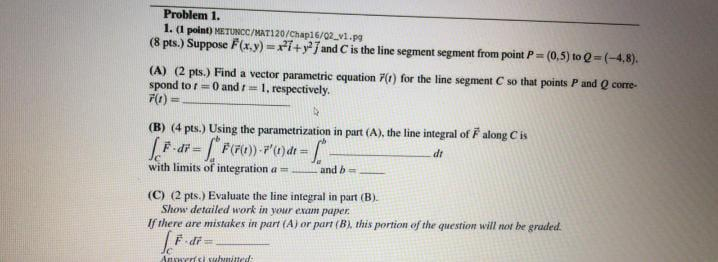### Create an Account

Home / Questions / Problem 1. 1. (1 point) METUNCC/MAT120/Chap16/02_v1.pg (8 pts.) Suppose F(x,y)=i+y; and C ...

# Problem 1. 1. (1 point) METUNCC/MAT120/Chap16/02_v1.pg (8 pts.) Suppose F(x,y)=i+y; and C is the line segment segment from point P= = (0,5) to Q=(-4,8). (A) (2 pts.) Find a vector parametric equation

Problem 1. 1. (1 point) METUNCC/MAT120/Chap16/02_v1.pg (8 pts.) Suppose F(x,y)=i+y; and C is the line segment segment from point P= = (0,5) to Q=(-4,8). (A) (2 pts.) Find a vector parametric equation (1) for the line segment C so that points P and corre- spond to t=0 and =1, respectively. 7(0) = (B) (4 pts. Using the parametrization in part (A), the line integral of along Cis [-di= {* F(F(t)-x't)dt = dt with limits of integration a = and b (C) (2 pts.) Evaluate the line integral in part (B). Show detailed work in your exam paper If there are mistakes in part (A) or part (B), this portion of the question will not be graded. Fidia Anici sunittedApr 28 2021 View more View LessSubscribe To Get Solution